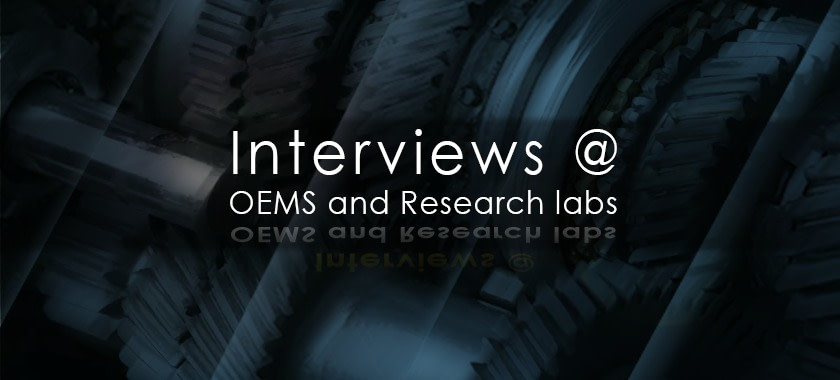# Interviews at OEMs and Research labs – Connecting Theory to PracticeAn OEM or a research interview is designed to test the ability of the candidate to solve a problem by applying physics-driven thinking skills. Unless the interviews are for people who would develop mechanical methods/mathematical models for high-end research interviews mostly check fundamentals, application and thinking skills.

### Entrant Level- Typical OEM

Expectation: Understanding of basic loads and ability to calculate stress in simple components

### Interviewer

As it could be observed in a composite beam the neutral axis is located in the stiffer member as it could support greater load. Could we relate this phenomenon to a vibration situation?

### Candidate

Analysis: Let us consider a two-mass system and the equivalent of neutral axis is the node which is characterised by zero displacements.

The node initially lies in the middle. However, as the left mass or mass moment of inertia increases, the node shifts to the left towards the heavier mass (node is a point of zero displacements and antinode is a point of maximum displacement).

### Entrant Level- Typical Research

Expectation: Understanding of basic loads and ability to observe unobvious facets

### Interviewer

Explain the discontinuity stress at the weld line where two different types of pressure cylinders are welded.

### Step 1

Observation: Both pressure vessels have growths that are different at weld line which leads to local bending.

This phenomenon is observed in many products where discontinuities of material temperature or thermal properties etc exist

### Engineers' note:

In many cases when two components grow mechanically to different extents, restraining each other at the joint where two components are joined, local bending is caused.

### Step 2 - Quantification

Consider a spherical pressure vessel welded to a cylindrical pressure vessel. At the joint, the spherical pressure vessel and cylindrical pressure vessels have different growths when subjected to internal pressure. The table below gives the radial growths of both the vessels, which is nothing, but hoop strain multiplied by the radius.

### Fairly experienced

Expectation: Critical thinking skills for product design

### Interviewer

Explain a first principle method to find the angular relationship between the gear teeth, of Gear 1 and Gear 2 assuming them to be of same module and that both gears have even teeth. Assume that the Gear axis is ideally located and has no eccentricity. Assume the Gear 2 to be the reference.

### Step 1

Observation: The Gear is held between the centers. The gear axis is established using the digital height gauge. The roller is kept on Gear1 and dialed to already set (center distance plus the radius of the roller) height and set the dial to zero. Shift the roller to the next gear’s (gear 1) corresponding tooth space and dial the roller. The indicator reading is the relative angular position error. Since both gears are of same module, the same roller can be used since module is a measure tooth thickness or tooth space.

### Step 2 - Candidate must quantify the problem understanding

If the center axis height is not set and one of the toot spaces is arbitrarily made approximately horizontal and readings are taken, then there can be a large error. The explanation, both graphical and mathematical, is given below.

The error is computed as follows: Taking r1 and r2 as the pitch radii, let the angular position error be ∆θ.  One roller axis kept in gear tooth space of Gear-1 is parallel to gear axis and hence error is contributed by only Gear-2.

### Step 3 - Analysis

• In many situations first principle based measurement becomes warranted
• This requires both mechanical visualisation and mathematical insight
• It is for batch production of complex components

### Did you know?

• Could you spot product situations where tolerancing errors (dimensional, geometric or surface characteristics including case depth) could bring about sever reduction in life of the components?
• List and discuss all the parameters for gear grinding that if addressed properly could augment the process tightness.

0
0